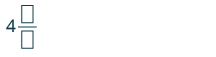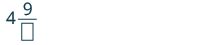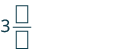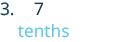## Converting Decimals to Fractions or Mixed Numbers

### Learning Outcomes

• Convert a decimal to a fraction or mixed number

We often need to rewrite decimals as fractions or mixed numbers. Let’s go back to our lunch order to see how we can convert decimal numbers to fractions. We know that $5.03$ means $5$ dollars and $3$ cents. Since there are $100$ cents in one dollar, $3$ cents means ${\Large\frac{3}{100}}$ of a dollar, so $0.03={\Large\frac{3}{100}}$.

We convert decimals to fractions by identifying the place value of the farthest right digit. In the decimal $0.03$, the $3$ is in the hundredths place, so $100$ is the denominator of the fraction equivalent to $0.03$.

$0.03={\Large\frac{3}{100}}$

For our $5.03$ lunch, we can write the decimal $5.03$ as a mixed number.

$5.03=5{\Large\frac{3}{100}}$

Notice that when the number to the left of the decimal is zero, we get a proper fraction. When the number to the left of the decimal is not zero, we get a mixed number.

### Convert a decimal number to a fraction or mixed number.

1. Look at the number to the left of the decimal.
• If it is zero, the decimal converts to a proper fraction.
• If it is not zero, the decimal converts to a mixed number.
• Write the whole number.
2. Determine the place value of the final digit.
3. Write the fraction.
• numerator—the ‘numbers’ to the right of the decimal point
• denominator—the place value corresponding to the final digit
4. Simplify the fraction, if possible.

### example

Write each of the following decimal numbers as a fraction or a mixed number:

1. $4.09$
2. $3.7$
3. $-0.286$

Solution:

 1. $4.09$ There is a $4$ to the left of the decimal point. Write “$4$” as the whole number part of the mixed number.Determine the place value of the final digit.Write the fraction. Write $9$ in the numerator as it is the number to the right of the decimal point.Write $100$ in the denominator as the place value of the final digit, $9$, is hundredth. $4{\Large\frac{9}{100}}$ The fraction is in simplest form. So, $4.09=4{\Large\frac{9}{100}}$

Did you notice that the number of zeros in the denominator is the same as the number of decimal places?

 2. $3.7$ There is a $3$ to the left of the decimal point. Write “$3$” as the whole number part of the mixed number.Determine the place value of the final digit.Write the fraction. Write $7$ in the numerator as it is the number to the right of the decimal point.Write $10$ in the denominator as the place value of the final digit, $7$, is tenths. $3{\Large\frac{7}{10}}$ The fraction is in simplest form. So, $3.7=3{\Large\frac{7}{10}}$
 3. $−0.286$ There is a $0$ to the left of the decimal point. Write a negative sign before the fraction.Determine the place value of the final digit and write it in the denominator.Write the fraction. Write $286$ in the numerator as it is the number to the right of the decimal point. Write $1,000$ in the denominator as the place value of the final digit, $6$, is thousandths. $-{\Large\frac{286}{1000}}$ We remove a common factor of $2$ to simplify the fraction. $-{\Large\frac{143}{500}}$

### try it

In the next video example, we who how to convert a decimal into a fraction.